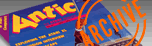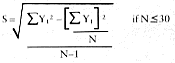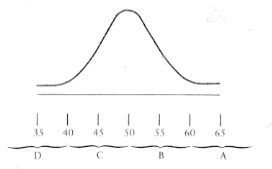`ANTIC VOL. 1, NO. 6 / FEBRUARY 1983`

## Education

by Clyde Spencer

The many ways to assign student's grades all seem to have their advantages and disadvantages. It is likely that the technique you use was originally adopted for its simplicity, speed and ease of use. Tossing the papers down the stairs and giving A's to the farthest even has some merit. However, a more professional approach might be desired.

Most approaches require additional effort and varying degrees of mathematical expertise, and the more rigorous, statistically-based ones have not been in favor.

Let's assume you are teaching a course where frequent standardized testing is appropriate and the students' abilities and accomplishments can be expected to be "normally distributed". If you plotted the numeric scores against the number of students that received scores within a given small interval, the plot would approximate the standard "Bell" shape. You should have at least 20 students minimum-a typical class size.

This approach also takes into account variations in the level of difficulty of your tests and a student's preparation. In your current grading system you may assign 70% as the breaking point between a "C" and a "D", and this will be much too subjective and totally unreasonable for some exams.

An important advantage of this method is that both you and the students can have a good idea of what a student's class standing is at any time. The student's grade is determined by adding together all his McCall's T scores and dividing by the number of scores. That average is then compared with the equivalent letter grades.

The McCall's T score will "normalize" all the scores and compare the class performance as a whole against the individual scores, not some predetermined absolute. If your mathematics is strong, you can verify the algebraic manipulations from the BASIC program listing which follows. For the rest of you, you must trust that the approach calculates the class average (mean) and standard deviation. The mean is then subtracted from the individual student's score and divided by 1/lOth the standard deviation. This gives a number between about -25 and + 25, with most near zero. The constant 50 is then added to be sure all the numbers are positive. That is your McCall's T score. Fifty percent of the students will always have scores greater than 50. Sixtyeight percent will have scores between 40 and 60 and better than ninety-five percent of all the students will have a score between 30 and 70.

If you've ever been exposed to statistics, those numbers should look vaguely familiar. They represent 1 and 2 standard deviations from the mean. If you wanted to give no more than 15% A's, you would see that about 15% of the area in a normal curve is found above a value of 1.04 standard deviations above the mean. That would translate into a McCall's T score of 60.4 (10 x 1.04 + 50). I have in the past adopted cutoffs of 30, 40, 50 and 60. That effectively gives 50% A's and B's which is in line with a community college average.

If I've convinced you to try something new, what follows are a Visicalc template and a less elegant BASIC program along with sample printouts. You will find the BASIC program easier to type in than the Visicalc template, but Visicalc is definitely the preferred way to go!

Your students, being human, will never have seen anything like this before and some will resist change. You will need to explain the procedure to them, and re-explain it. If you think the method has merit, hang in there, but expect and be prepared for complaints. Just as there is no perfect form of government, these is no perfect grading system!

All grade scores will be reported in terms of McCall's T score as well as the raw score.

McCall's T score is defined as follows: T=50+10Z, where Z=(Y1-Y)/S andS=sample standard deviation
Y1=student's raw score
Y=class mean
N=total sample number
therefore T=50+10(Y1-Y)/S```COURSE: TYPING 001            DATE: 14 FEB. 1983
QUIZ #1

STUDENT NAME      RAW SCORE            "T" SCORE
Abbey, James       42                   58.79
Blake, John        34                   51.37
Castro, Jessee     49                   65.29
Dalton, Maria      24                   42.09
Ellis, Mark        35                   52.30
Felix, Gloria      26                   43.95
Gallo, Joe         30                   47.66
Hanes, Sam         22                   40.24
Ivanovich, William 41                   57.86
Jackson, Anthony   40                   56.94
Kaufman, David     31                   48.59
Lang, Mary         47                   63.43
Malone, Kathy      22                   40.24
Nguyen, Kim        32                   49.51
O'Neal, Doug       12                   30.96
Perez, Delores     25                   43.02
Reddy, Barbara     18                   36.52
Sanford, Tom       41                   57.86
Takahashi, Isao    41                   57.86
Unger, Max         50                   66.21
----------------------------------------------
TOTAL              683
NUMBER              21
AVERAGE (MEAN)      32.52
STAN. DEV           10.78
HIGH                50
LOW                 12
```

[CODE]